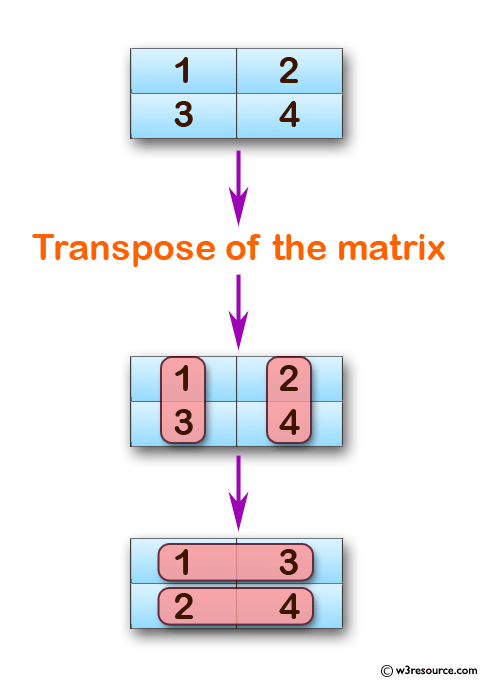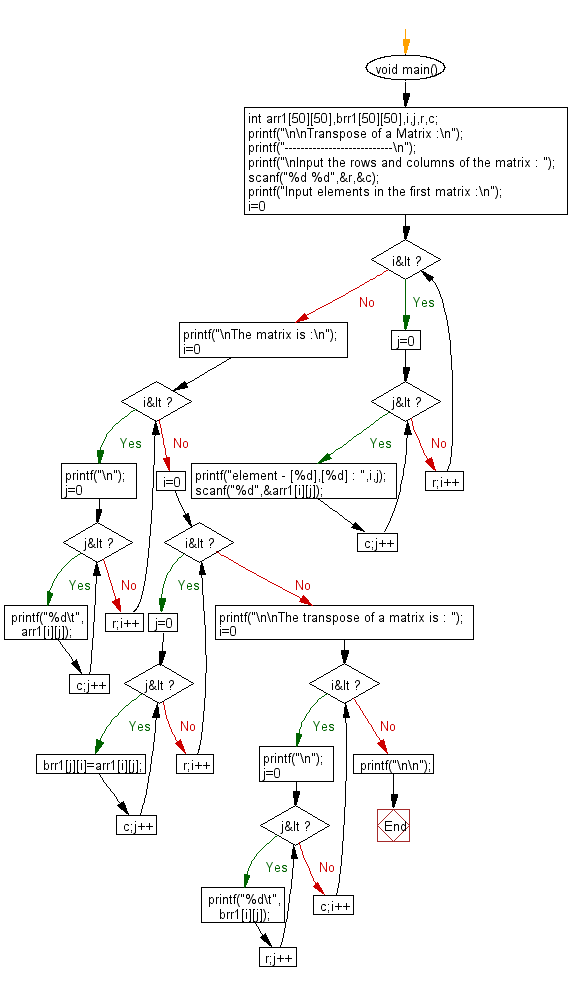﻿ C exercises: Transpose of a Matrix - w3resource# C Exercises: Transpose of a Matrix

## C Array: Exercise-22 with Solution

Write a program in C to find transpose of a given matrix.

Pictorial Presentation:Sample Solution:

C Code:

``````#include <stdio.h>

void main()

{
int arr1,brr1,i,j,r,c;

printf("\n\nTranspose of a Matrix :\n");
printf("---------------------------\n");

printf("\nInput the rows and columns of the matrix : ");
scanf("%d %d",&r,&c);

printf("Input elements in the first matrix :\n");
for(i=0;i<r;i++)
{
for(j=0;j<c;j++)
{
printf("element - [%d],[%d] : ",i,j);
scanf("%d",&arr1[i][j]);
}
}

printf("\nThe matrix is :\n");
for(i=0;i<r;i++)
{
printf("\n");
for(j=0;j<c;j++)
printf("%d\t",arr1[i][j]);
}

for(i=0;i<r;i++)
{
for(j=0;j<c;j++)
{
brr1[j][i]=arr1[i][j];
}
}

printf("\n\nThe transpose of a matrix is : ");
for(i=0;i<c;i++){
printf("\n");
for(j=0;j<r;j++){
printf("%d\t",brr1[i][j]);
}
}
printf("\n\n");
}
```
```

Sample Output:

```Transpose of a Matrix :
---------------------------

Input the rows and columns of the matrix : 2 2
Input elements in the first matrix :
element - , : 1
element - , : 2
element - , : 3
element - , : 4

The matrix is :

1       2
3       4

The transpose of a matrix is :
1       3
2       4
```

Flowchart:C Programming Code Editor:

Improve this sample solution and post your code through Disqus.

What is the difficulty level of this exercise?

﻿

## C Programming: Tips of the Day

Where is the C auto keyword used?

auto is a modifier like static. It defines the storage class of a variable. However, since the default for local variables is auto, you don't normally need to manually specify it.

Ref : https://bit.ly/3yzwC9r